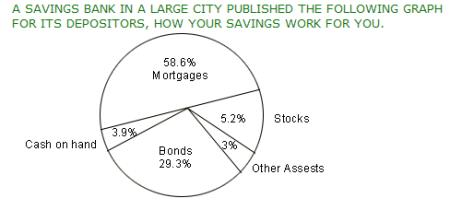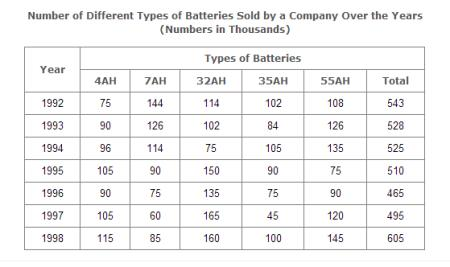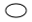GRE Quantitative Reasoning Questions

100 Data Interpretation Questions (Set 8)

 Select Exercise Lists

By Question Type

By Content

 Selected Set: 8
Select Exercise Set:Select Question:

1. About how many degrees (to the nearest degree) are in the angle of the sector representing mortgages?59106211246318
2. The annual rate of interest from "other assets" is 4.8%. If the total assets of the bank are 57.6 million dollars, what is the annual income (in dollars) from "other assets"?82,944921,6001,728,0002,764,8003,600,000
3. The average annual interest on mortgage investments is m% and the average annual interest on the mortgage bond investment is b%. If the annual interest on the bond investment is x dollars, how many dollars are invested in mortgages?xm/b.xb/m.100xb/mbx/100m.200x/b
4. About how many degrees (to the nearest degree) are in the angle of the sector representing cash on hand?1141414039321
5. What was the approximate percentage increase in the sales of 55AH batteries in 1998 compared to that in 1992?28%31%33%34%
6. The total sales of all the seven years is the maximum for which battery?4AH7AH32AH35AH
7. What is the difference in the number of 35AH batteries sold in 1993 and 1997?24000280003500039000
8. The percentage of 4AH batteries sold to the total number of batteries sold was maximum in the year?1994199519961997
9. In case of which battery there was a continuous decrease in sales from 1992 to 1997?4AH7AH32AH35AH